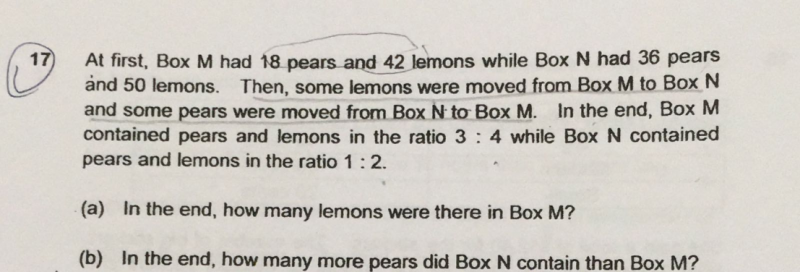# QuestionHi,

Need help on P6 question. Appreciate it for all the help in advance.

Regards.

Source: Nanyang Primary

(a)
At first —
Box M —
pears : 18
lemons : 42

Box N —
pears : 36
lemons : 50

In the end —
Box M —
pears : 3u
lemons : 4u

Box N —
pears : 1p
lemons : 2p

3u + 1p ——-> 18 + 36 = 54
6u + 2p ——-> 108
4u + 2p ——-> 42 + 50 = 92
2u ——-> 16
1u ——-> 8
4u ——-> 32

(b)
3 x 8 + 1p = 24 + 1p ——-> 54
1p ——- 54 – 24 = 30
3u ——-> 24
30 – 24 = 6

Ans : (a) 32 lemons; (b) 6 more pears.

0 Replies 0 Likes

This is a Matrix question.

1.  Pear = 18 + 36 = 54.   Lemon = 42 + 50 = 92

2.  Box M Pear 3u, Lemon 4u

 Box M Box N total Pear 3u 54 – 3u 54 Lemon 4u 92 – 4u 92

(54 – 3u)/1  = (92 – 4u) /2

108 – 6u = 92 – 4u

108 – 92 = 6u – 4u

2u = 16

1u = 16 ÷ 2 =8

a)

4u = 8 × 34= 32

b)

3u = 3 × 8 = 24

54 – 24 = 30

30 – 24 = 6 more pears

1 Reply 0 Likes

Hi Mr Khong,

Thank you for your prompt response.

However, there is something that I don’t understand about this statement:

(54 – 3u)/1  = (92 – 4u) /2

Why do we to divide by 2?

Thanks.

0 Replies 0 Likes